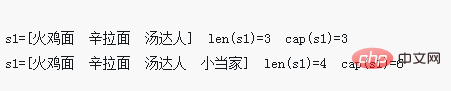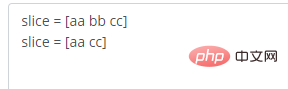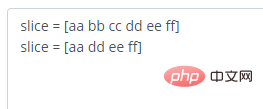# go语言中切片怎么增删元素

go语言中可用append()为切片动态增加和删除元素。增加元素的语法“slice = append(slice,elem1,elem2)”。删除元素可分两种：1、删除索引处的元素，语法“slice=append(slice[:i],slice[i+1:]...)”；2、删除指定索引间的元素，语法“slice=append(slice[:i],slice[i2:]...)”。`slice = append(slice,elem1,elem2)`
• append括号内，第一个参数slice后可以加多个参数。

```package  main
import "fmt"
//切片进阶操作

func main(){
//append()为切片追加元素
s1 := []string {"火鸡面","辛拉面","汤达人"}
fmt.Printf("s1=%v len(s1)=%d cap(s1)=%d\n",s1,len(s1),cap(s1))

//调用append函数必须用原来的切片变量接收返回值
s1 = append(s1,"小当家") //append追加元素，原来的底层数组装不下的时候，Go就会创建新的底层数组来保存这个切片
fmt.Printf("s1=%v len(s1)=%d cap(s1)=%d\n",s1,len(s1),cap(s1))//cap增加两倍
}```Go 语言 的 切片 删除元素也可以使用 append 函数 来间接的实现。

`slice = append(slice[:index], slice[index+1:]...)`

slice要删除元素的切片。
index要删除的索引。

`slice = append(slice[:index], slice[index2:]...)`

slice要删除元素的切片。
index要删除的开始索引。
index2要删除的结束索引。

```package main
import (
"fmt"
)
func main() {
//使用append函数，实现删除切片 index 处的元素
var slice = []string{"aa", "bb", "cc"}
fmt.Println("slice =", slice)
slice = append(slice[:1], slice[2:]...)
fmt.Println("slice =", slice)
}``````package main
import (
"fmt"
)
func main() {
//使用append函数，实现删除切片 index 处的元素
var slice = []string{"aa", "bb", "cc", "dd", "ee", "ff"}
fmt.Println("slice =", slice)
slice = append(slice[:1], slice[3:]...)
fmt.Println("slice =", slice)
}```【相关推荐：Go视频教程编程教学1/1